# Unit 5 Chemical Reactions I Intro to Reactions

• Slides: 38Unit 5 – Chemical Reactions I. Intro to Reactions I II IVSigns of Chemical Reactions There are five main signs that indicate a chemical reaction has taken place: release input change in color change in odor production of new gases or vapor input or release of energy difficult to reverse ALSO, formation of precipitate (ppt) indicates a rxn!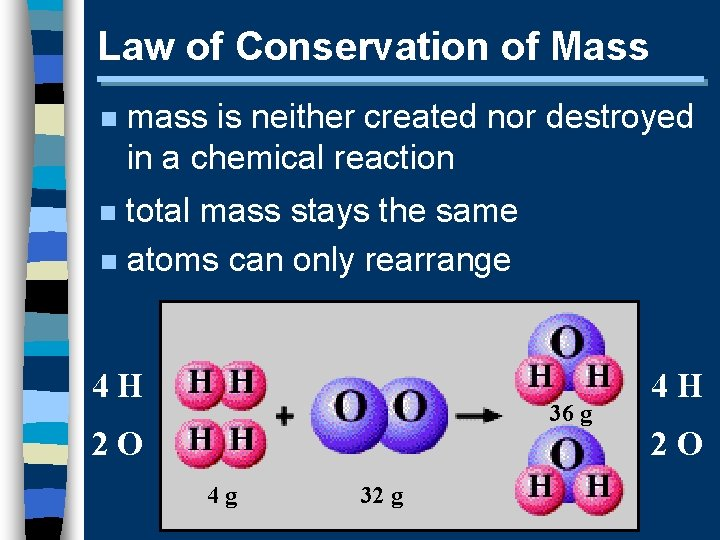Law of Conservation of Mass n mass is neither created nor destroyed in a chemical reaction total mass stays the same n atoms can only rearrange n 4 H 36 g 2 O 4 g 32 g 4 H 2 OChemical Equations A+B C+D REACTANTS PRODUCTS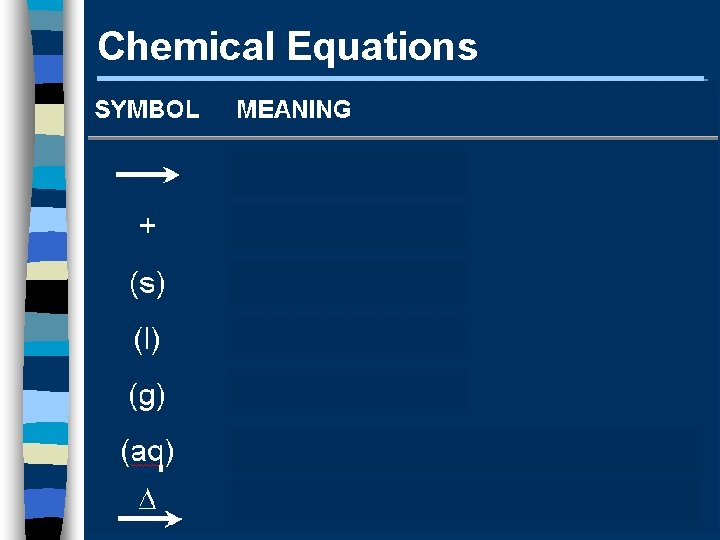Chemical Equations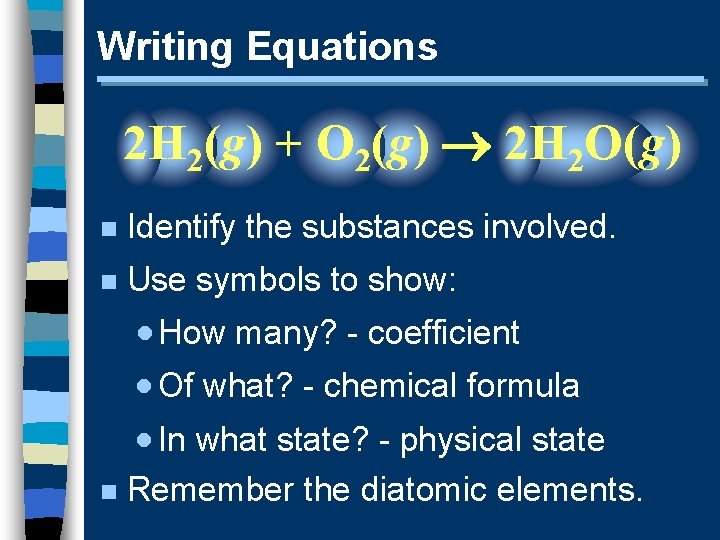Writing Equations 2 H 2(g) + O 2(g) 2 H 2 O(g) n Identify the substances involved. n Use symbols to show: · How many? - coefficient · Of what? - chemical formula · In what state? - physical state n Remember the diatomic elements.Writing Equations Two atoms of aluminum react with three units of aqueous copper(II) chloride to produce three atoms of copper and two units of aqueous aluminum chloride. • How many? • Of what? • In what state? 2 Al(s) + 3 Cu. Cl 2(aq) 3 Cu(s) + 2 Al. Cl 3(aq)Describing Equations n Describing Coefficients: · individual atom of an element = “atom” · covalent substance = “molecule” · ionic substance = “formula unit” 3 CO 2 3 molecules of carbon dioxide 2 Mg 2 atoms of magnesium 4 Mg. O 4 form. units of magnesium oxide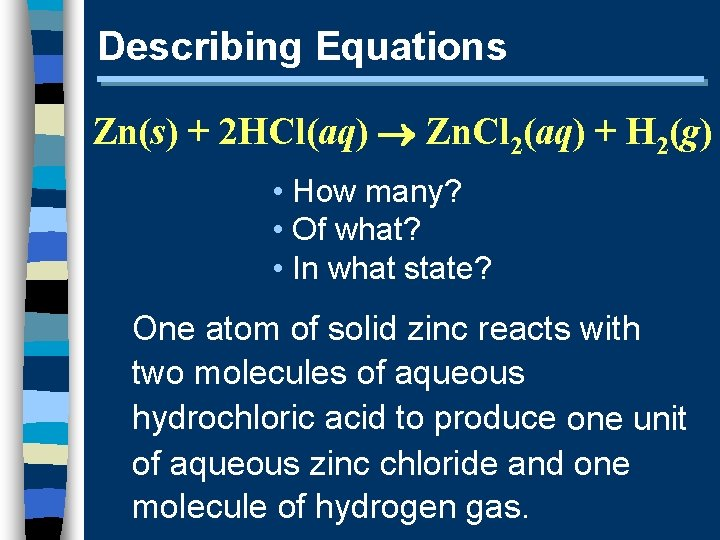Describing Equations Zn(s) + 2 HCl(aq) Zn. Cl 2(aq) + H 2(g) • How many? • Of what? • In what state? One atom of solid zinc reacts with two molecules of aqueous hydrochloric acid to produce one unit of aqueous zinc chloride and one molecule of hydrogen gas.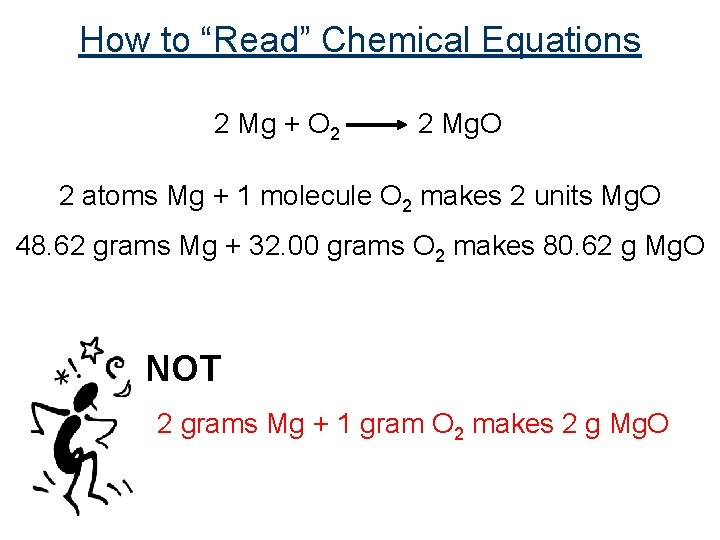How to “Read” Chemical Equations 2 Mg + O 2 2 Mg. O 2 atoms Mg + 1 molecule O 2 makes 2 units Mg. O 48. 62 grams Mg + 32. 00 grams O 2 makes 80. 62 g Mg. O NOT 2 grams Mg + 1 gram O 2 makes 2 g Mg. O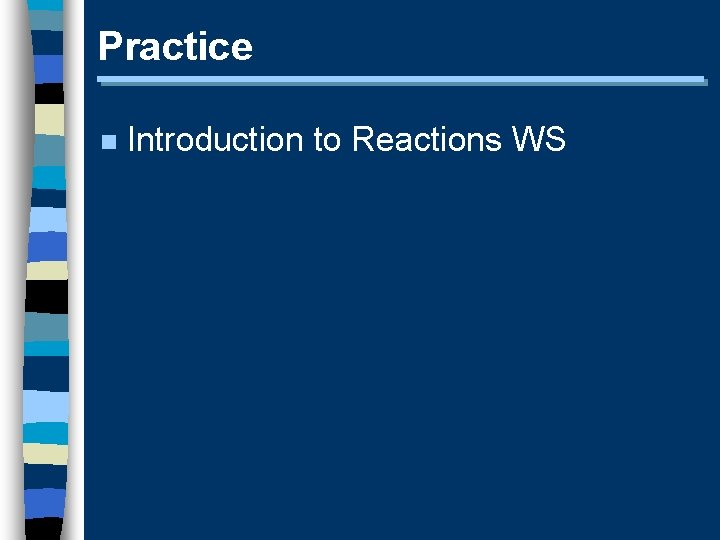Practice n Introduction to Reactions WSUnit 5 – Chemical Reactions II. Balancing Equations I II IV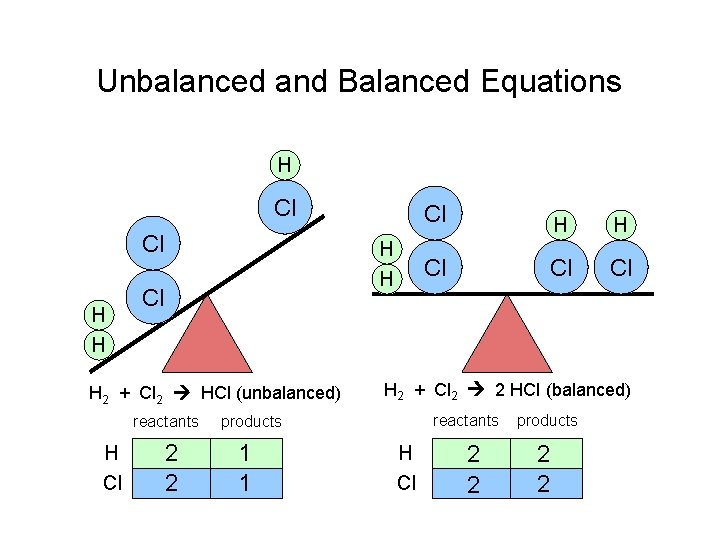Unbalanced and Balanced Equations H Cl Cl H H Cl H 2 + Cl 2 HCl (unbalanced) reactants H Cl 2 2 H H Cl Cl Cl H 2 + Cl 2 2 HCl (balanced) reactants products 1 1 Cl H Cl 2 2 products 2 2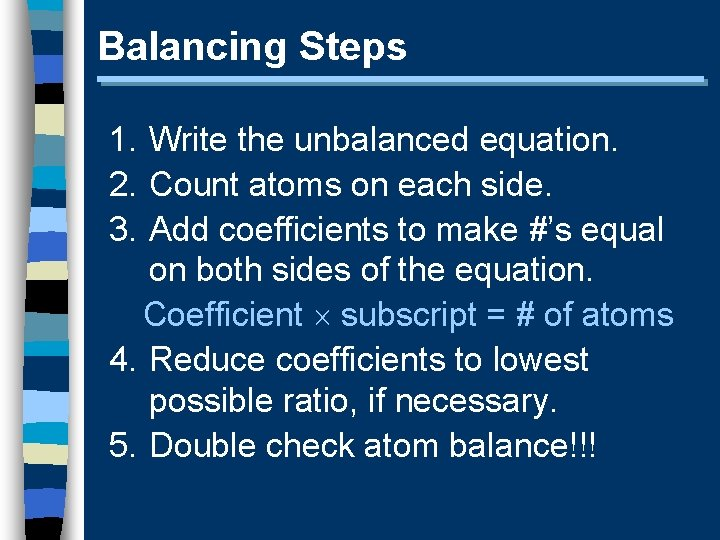Balancing Steps 1. Write the unbalanced equation. 2. Count atoms on each side. 3. Add coefficients to make #’s equal on both sides of the equation. Coefficient subscript = # of atoms 4. Reduce coefficients to lowest possible ratio, if necessary. 5. Double check atom balance!!!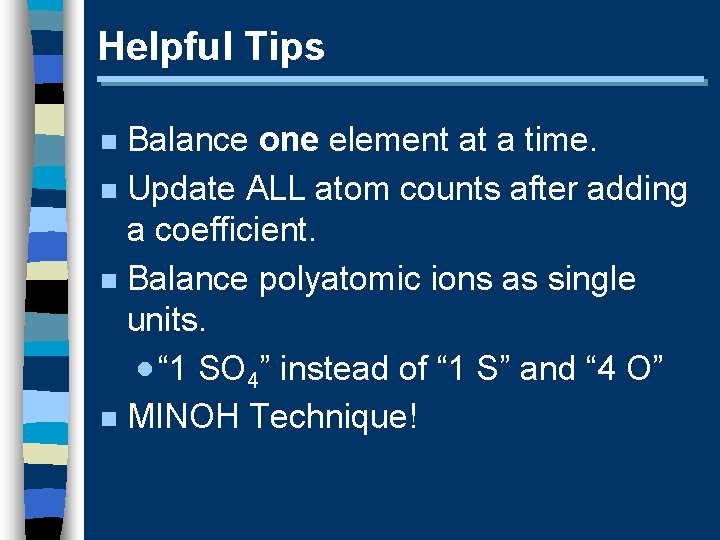Helpful Tips Balance one element at a time. n Update ALL atom counts after adding a coefficient. n Balance polyatomic ions as single units. · “ 1 SO 4” instead of “ 1 S” and “ 4 O” n MINOH Technique! n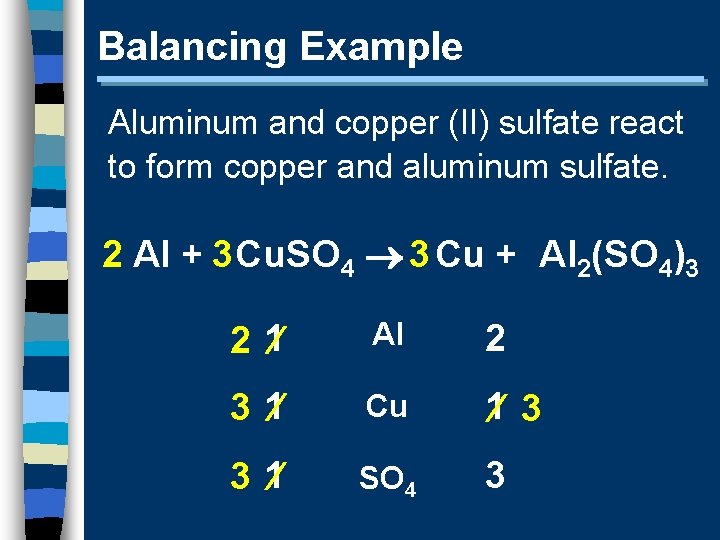Balancing Example Aluminum and copper (II) sulfate react to form copper and aluminum sulfate. 2 Al + 3 Cu. SO 4 3 Cu + Al 2(SO 4)3 2 1 Al 2 3 1 Cu 1 3 3 1 SO 4 3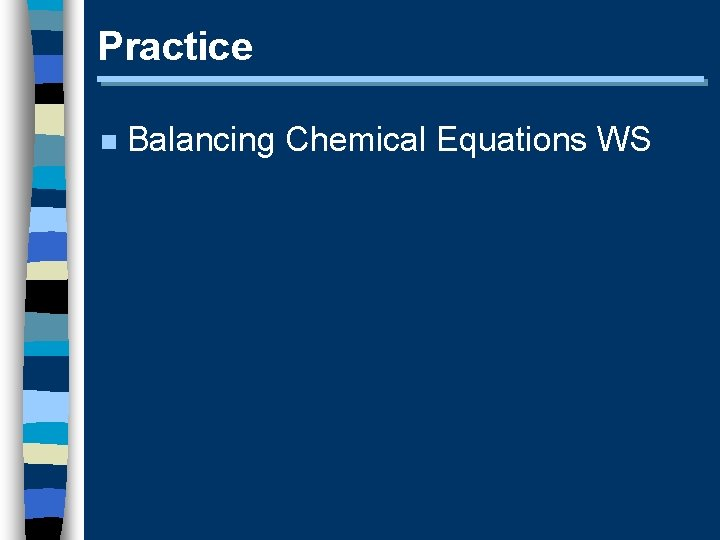Practice n Balancing Chemical Equations WS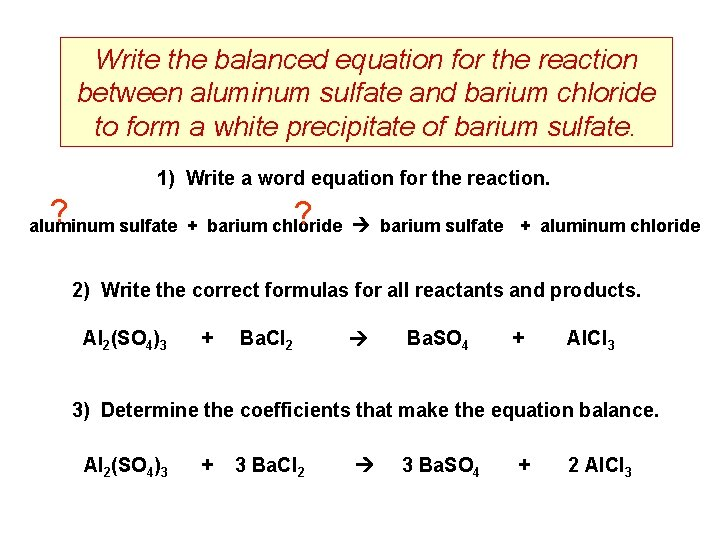Write the balanced equation for the reaction between aluminum sulfate and barium chloride to form a white precipitate of barium sulfate. 1) Write a word equation for the reaction. ? ? aluminum sulfate + barium chloride barium sulfate + aluminum chloride 2) Write the correct formulas for all reactants and products. Al 2(SO 4)3 + Ba. Cl 2 Ba. SO 4 + Al. Cl 3 3) Determine the coefficients that make the equation balance. Al 2(SO 4)3 + 3 Ba. Cl 2 3 Ba. SO 4 + 2 Al. Cl 3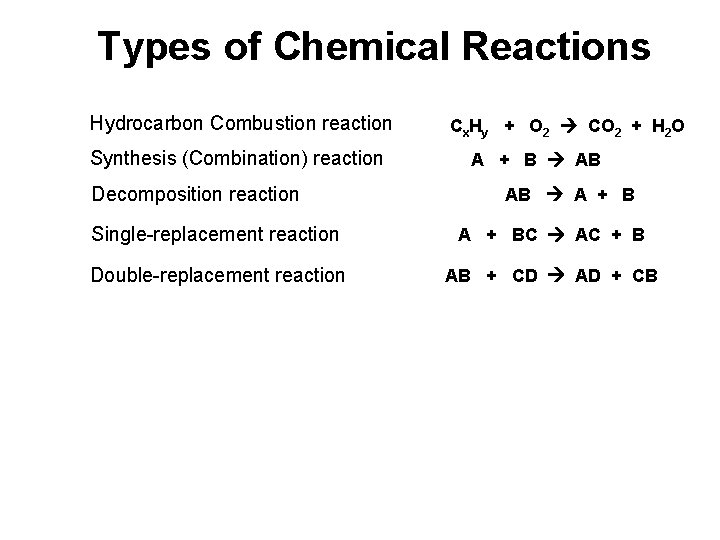Types of Chemical Reactions Hydrocarbon Combustion reaction Synthesis (Combination) reaction Decomposition reaction Cx. Hy + O 2 CO 2 + H 2 O A + B AB AB A + B Single-replacement reaction A + BC AC + B Double-replacement reaction AB + CD AD + CBUnit 5 – Chemical Reactions III. Types of Chemical Reactions I II IVHydrocarbon Combustion n the burning of a compound containing carbon and hydrogen in O 2 to produce heat CH + O 2 CO 2 + H 2 O CH 4(g) +2 O 2(g) CO 2(g) +2 H 2 O(g)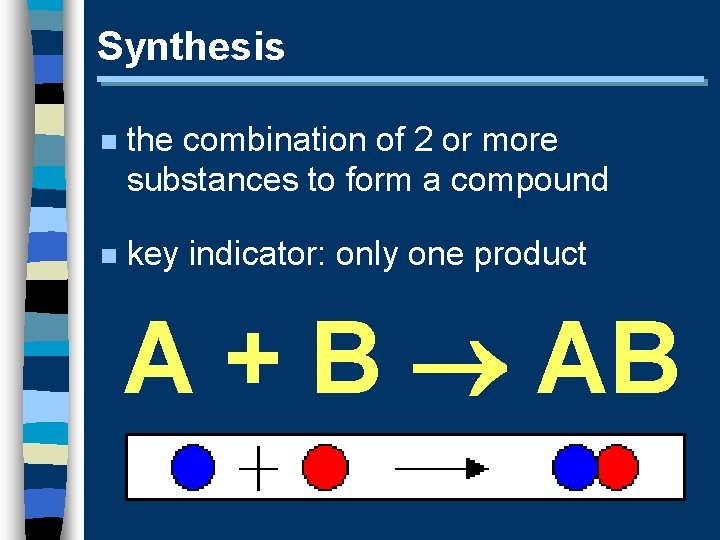Synthesis n the combination of 2 or more substances to form a compound n key indicator: only one product A + B ABSynthesis n Predicting Products: · ionic - cancel charges · covalent - hard to tell 2 Al(s)+ 3 Cl 2(g) 2 Al. Cl 3(s)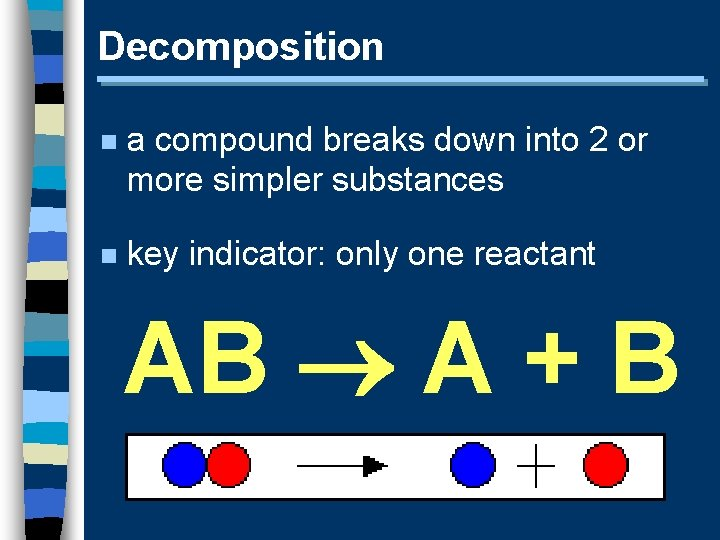Decomposition n a compound breaks down into 2 or more simpler substances n key indicator: only one reactant AB A + B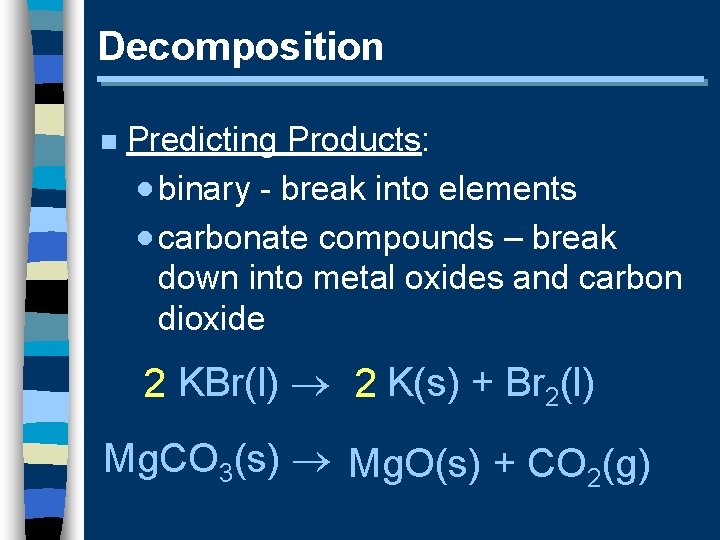Decomposition n Predicting Products: · binary - break into elements · carbonate compounds – break down into metal oxides and carbon dioxide 2 KBr(l) 2 K(s) + Br 2(l) Mg. CO 3(s) Mg. O(s) + CO 2(g)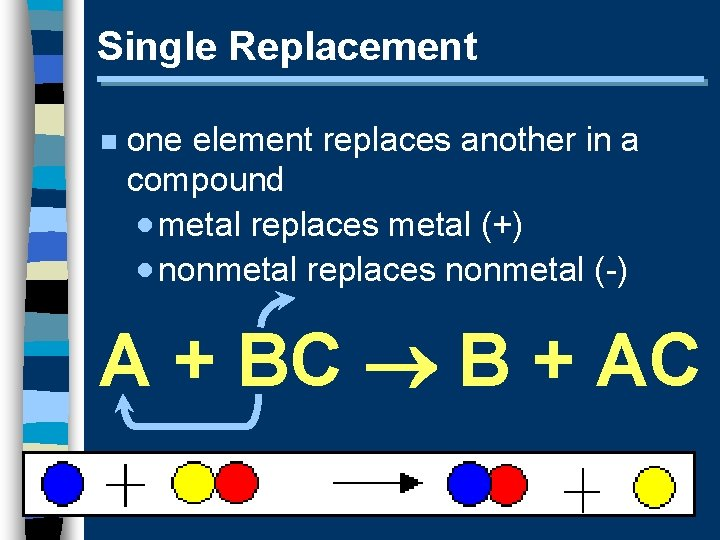Single Replacement n one element replaces another in a compound · metal replaces metal (+) · nonmetal replaces nonmetal (-) A + BC B + ACSingle Replacement n Predicting Products: · metal (+) · nonmetal (-) · free element must be more active (check activity series) Fe(II) (s)+ Cu. SO 4(aq) Cu(s)+ Fe. SO 4(aq) Br 2(l)+ Na. Cl(aq) N. R.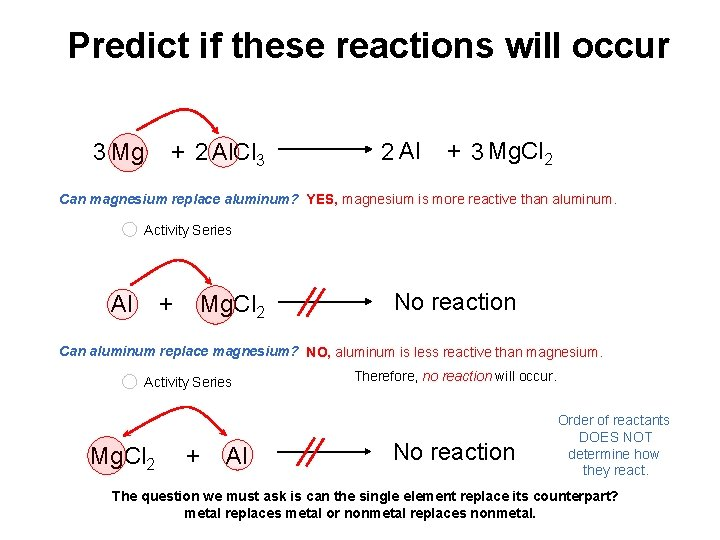Predict if these reactions will occur 3 Mg + 2 Al. Cl 3 2 Al + 3 Mg. Cl 2 Can magnesium replace aluminum? YES, magnesium is more reactive than aluminum. Activity Series Al + Mg. Cl 2 No reaction Can aluminum replace magnesium? NO, aluminum is less reactive than magnesium. Activity Series Mg. Cl 2 + Al Therefore, no reaction will occur. No reaction Order of reactants DOES NOT determine how they react. The question we must ask is can the single element replace its counterpart? metal replaces metal or nonmetal replaces nonmetal.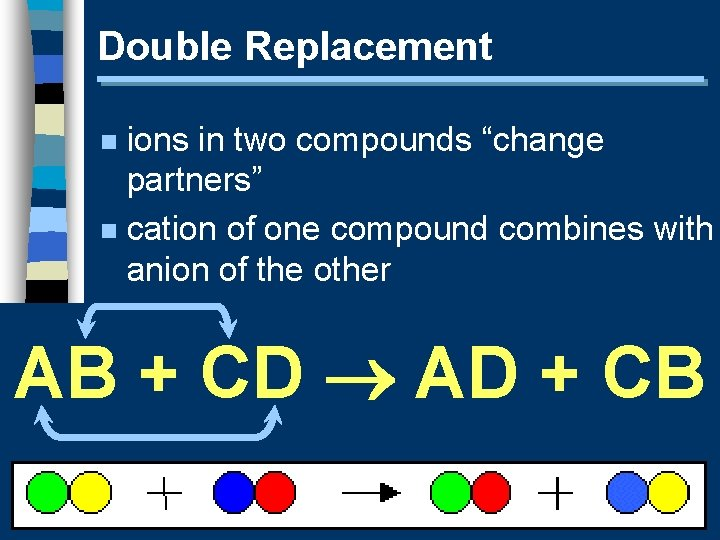Double Replacement ions in two compounds “change partners” n cation of one compound combines with anion of the other n AB + CD AD + CB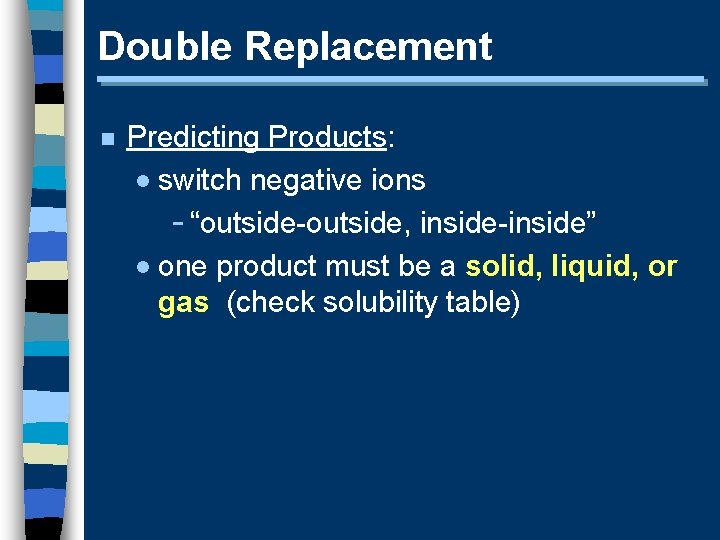Double Replacement n Predicting Products: · switch negative ions - “outside-outside, inside-inside” · one product must be a solid, liquid, or gas (check solubility table)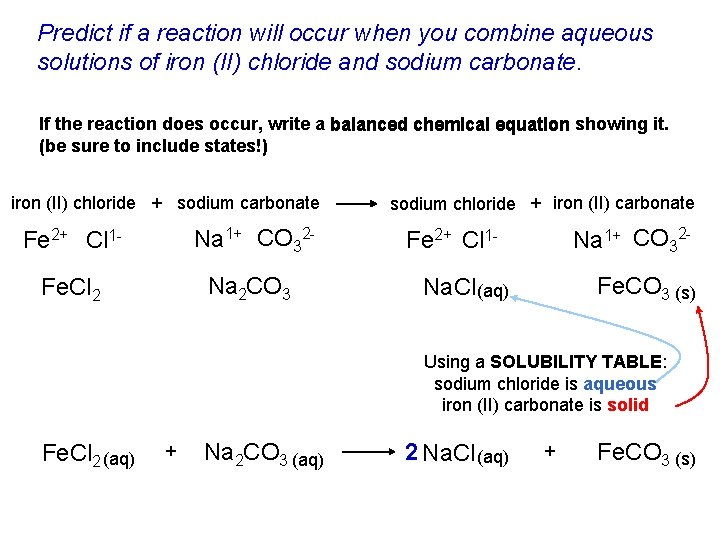Predict if a reaction will occur when you combine aqueous solutions of iron (II) chloride and sodium carbonate. If the reaction does occur, write a balanced chemical equation showing it. (be sure to include states!) iron (II) chloride + sodium carbonate Fe 2+ Cl 1 - Na 1+ CO 32 - Fe. Cl 2 Na 2 CO 3 sodium chloride + iron (II) carbonate Na 1+ CO 32 - Fe 2+ Cl 1 - Fe. CO 3 (s) Na. Cl (aq) Using a SOLUBILITY TABLE: sodium chloride is aqueous iron (II) carbonate is solid Fe. Cl 2 (aq) + Na 2 CO 3 (aq) 2 Na. Cl (aq) + Fe. CO 3 (s)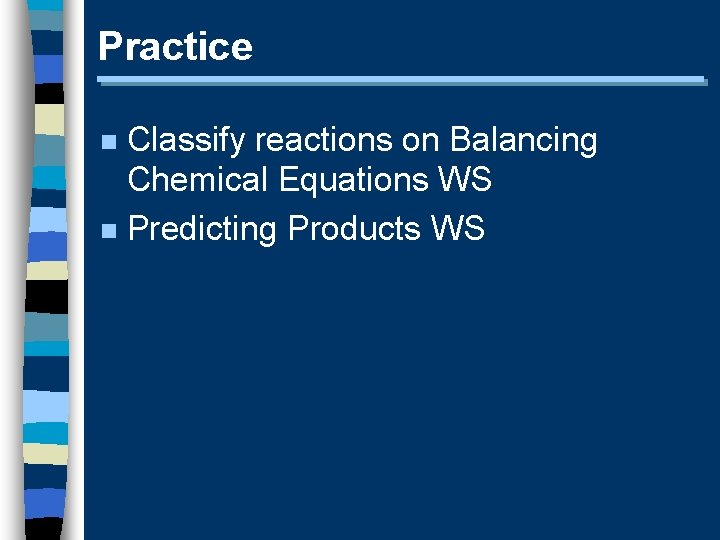Practice Classify reactions on Balancing Chemical Equations WS n Predicting Products WS n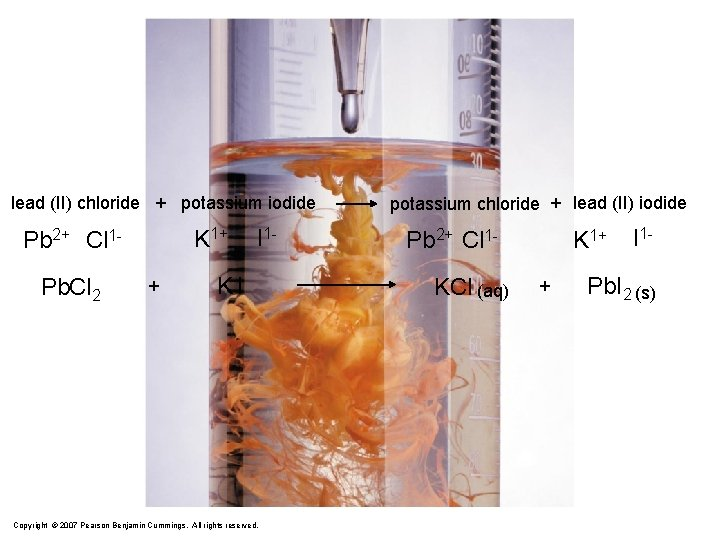lead (II) chloride + potassium iodide K 1+ Pb 2+ Cl 1 Pb. Cl 2 + I 1 - KI Copyright © 2007 Pearson Benjamin Cummings. All rights reserved. potassium chloride + lead (II) iodide Pb 2+ Cl 1 KCl (aq) K 1+ + I 1 - Pb. I 2 (s)lead (II) chloride + potassium iodide K 1+ Pb 2+ Cl 1 Pb. Cl 2 + I 1 - KI Copyright © 2007 Pearson Benjamin Cummings. All rights reserved. potassium chloride + lead (II) iodide Pb 2+ Cl 1 KCl (aq) K 1+ + I 1 - Pb. I 2 (s)Describing Precipitation Reactions n Molecular Equation · reactants and products are shown as compounds n Complete Ionic Equation · all aqueous substances are shown as ions n Net Ionic Equation · shows only the components that actually react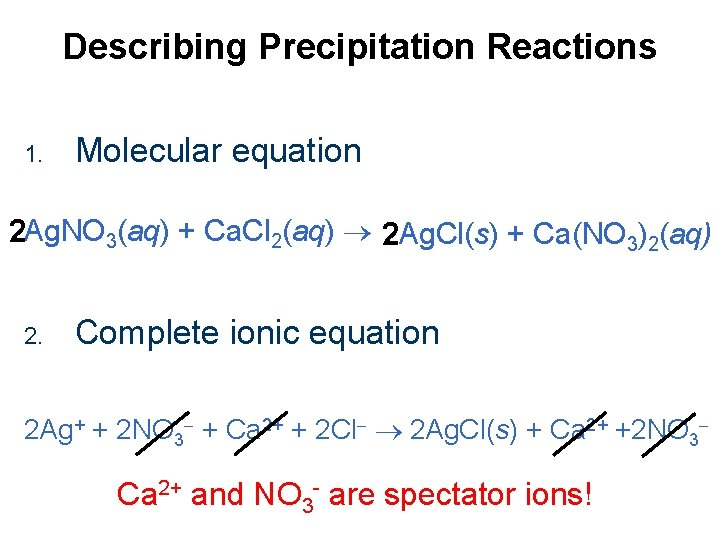Describing Precipitation Reactions 1. Molecular equation 2 Ag. NO 3(aq) + Ca. Cl 2(aq) 2 Ag. Cl(s) + Ca(NO 3)2(aq) 2. Complete ionic equation 2 Ag+ + 2 NO 3 + Ca 2+ + 2 Cl 2 Ag. Cl(s) + Ca 2+ +2 NO 3 Ca 2+ and NO 3 - are spectator ions!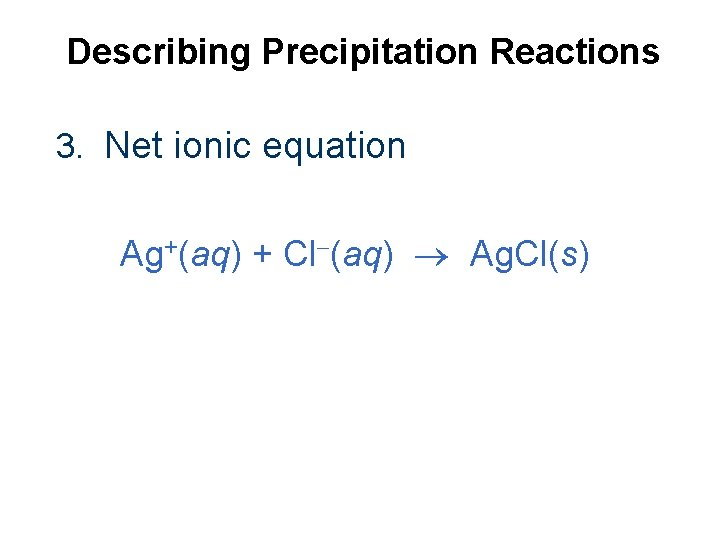Describing Precipitation Reactions 3. Net ionic equation Ag+(aq) + Cl (aq) Ag. Cl(s)Identify the spectator ions and write a net ionic equation when an aqueous solution of aluminum sulfate is mixed with aqueous ammonium hydroxide. Al 3+ SO 42 - aluminum sulfate + NH 41+ OH 1 - Al 3+ OH 1 - ammoniumhydroxide aluminum hydroxide + NH 41+ ammonium sulfate Al 2(SO 4)3(aq) + 6 NH 4 OH(aq) 2 Al(OH)3 (s) + 3 (NH 4)2 SO 4(aq) 2 Al 3+ + 3 SO 42 - + 6 NH 41+ + 6 OH 1 - 2 Al(OH)3(s) + 6 NH 41+ + 3 SO 42“spectator ions” 2 Al 3+(aq) + 6 OH 1 -(aq) 2 Al(OH)3(s) Net Ionic Equation SO 42 - Al 3+(aq) + 3 OH 1 -(aq) Al(OH)3(s)